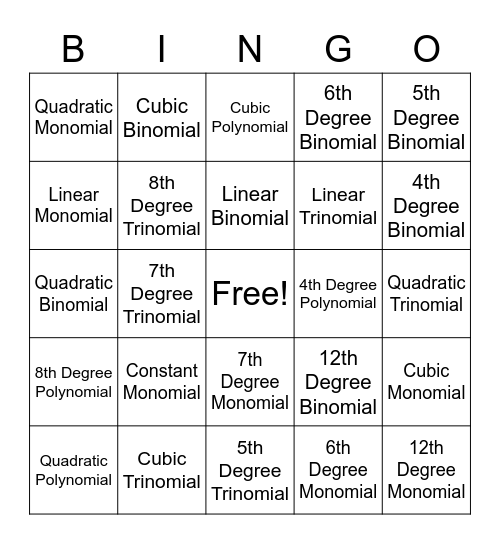# Classifying PolynomialsThis bingo card has a free space and 24 words: Constant Monomial, Cubic Trinomial, Linear Binomial, Quadratic Polynomial, 5th Degree Binomial, Quadratic Monomial, Linear Monomial, Cubic Binomial, 4th Degree Polynomial, 7th Degree Monomial, Linear Trinomial, Quadratic Binomial, 12th Degree Binomial, Cubic Monomial, 8th Degree Trinomial, 6th Degree Monomial, Quadratic Trinomial, Cubic Polynomial, 4th Degree Binomial, 7th Degree Trinomial, 12th Degree Monomial, 8th Degree Polynomial, 6th Degree Binomial and 5th Degree Trinomial.

## Play Online